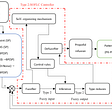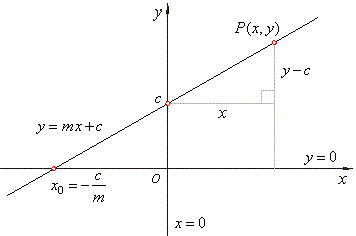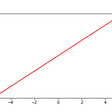# PyTorch 101: Linear regression with PyTorch

Even though Linear Regression is a problem that is probably better solved by other machine learning techniques such as Support Vector Machines and the like, it is still a crucial piece to understand in order to build more complicated models over time.

Linear regression can be defined as a way to understand the linear relationship between two values, X and Y. In this post we will train a model to understand the hidden linear function in our data and predict Y given X for the function `y = 3x + 4`, illustrated below:

## Background:

The function `y = 3x + 4` is just a random function we made up for demonstration purposes and any linear function you choose will suffice!

PyTorch, the super duper deep learning package, has a type of Layer known as a Linear layer, and just as its name suggests, it is useful for finding the linear mapping between any given input X and the label Y.

We are going to build a Feed Forward Neural Network with only ONE layer and one neuron. (This is not a deep neural network, those guys have more than 1 hidden layer, making a total of more than 3 layers)

Reminder: (Feed Forward) Neural Networks learn by passing an input through its hidden layers all the way to the end and their results are compared to the expected output to determine how well it performed. Each layer inside a Neural Network consists of neurons - these are the guys that actually do the learning. They each learn the function `y = f(x)`, where `f(x)` is the linear function that gives you Y when you give it X. The more layers you have the more you can learn. If you have 3 layers you can learn the function `h(g(f(x)))` where collectively, you have now learned the more advanced function h of g of f of x.

## Show me the code!

I use Jupyter Notebooks for quick iteration and if you don’t yet, I highly recommend you check it out.

First and foremost, import the modules we are going to use:

`import torchfrom torch.autograd import Variablefrom torch.nn import Linear, Module, MSELossfrom torch.optim import SGDimport numpy as np`

Above we have imported the PyTorch module (torch) and also imported some handy tools contained within it. To learn more about some of these “tools” please read my earlier post: Learning a quadratic equation with PyTorch: Intro to PyTorch

Two things to note:

1. We are going to use the Mean Squared Error Loss to calculate our loss
2. We will use Stochastic Gradient Descent to optimize our neural network

Now we create a function that will generate our data points:

`def generate_dataset(size = 100):    # equation: y = 3x + 4    inputs = []    outputs = []    for ix in range(size):        random_number = np.random.randint(100) / 100        inputs.append([random_number])        outputs.append([3 * random_number + 4])    return inputs, outputs`

In the snippet above, we declare our function `generate_dataset` which takes a size input for how many data samples to produce, and this function returns 2 data samples that model the equation `y = 3x + 4`. The function returns 2 arrays, one with the X values and one with the Y values.

Note: its important to scale the data to a value between 0 and 1, this is to make it easy for the neural network to learn since the numbers are much smaller. i.e: if we are using a random point between 0 and 100, as we are, we would then divide the random number by 100 so that it is well scaled.

You might be wondering why we generate our own data, its not (exactly) cheating, but we need to have some data to train our model on and if facing a real life problem with possibly linear properties, the same concepts will transfer easily. Examples of real life problems includes predicting what your grade will be given how many hours you study, predicting the gender of a person given just their height and/or weight, and many more!

## Define the Linear Regression model:

`class LinearRegressionModel(torch.nn.Module):    def __init__(self):        super(LinearRegressionModel, self).__init__()        self.fc = Linear(1, 1)        def forward(self, x):        x = self.fc(x)        return xmodel = LinearRegressionModel()`

The model is defined in the form of a class and inside this class we define 2 function/methods. The `__init__` function serves to initiate the class and preassign it some methods and variables, while the `forward` function defines the forward pass containing the manipulation of data as it makes its way from the input to the output.

Looking at the `forward` method, we see it only uses the one fully connected layer `self.fc1` that takes in one value and outputs another.

## Define the loss function & optimizer algorithm:

`# define critereon - loss functioncritereon = MSELoss()# define optimizeroptimizer = SGD(model.parameters(), lr=0.01, momentum=0.01)`

As we said earlier, we will be using the Mean Squared Error Loss function to calculate how “wrong” our neural networks predictions are, and we will use the Stochastic Gradient Descent algorithm to make improvements to our neural network so that we can get a better prediction next time around.

## Start training the network:

`inputs, labels = generate_dataset(100)nb_epochs = 30for epoch in range(nb_epochs):    epoch_loss = 0    for ix, x in enumerate(inputs):        # here x is the input. i.e. the input value of x        # and y_train[ix] is the output. i.e. y = f(x) = 3x + 4        y_pred = model(Variable(torch.Tensor(x)))                loss = critereon(y_pred, Variable(torch.Tensor(labels[ix])))                epoch_loss = loss.data                optimizer.zero_grad()        loss.backward()        optimizer.step()    print("Epoch {} : Loss {}".format(epoch, epoch_loss))`

The above code might seem daunting at first glance but I assure you, with just a basic understanding of python, its really easy to understand what is going on.

We start by generating only 100 examples to train our network, followed by explicitly defining the number of times we want to train our network on the 10 examples, otherwise known as the number of epochs.

We then start a loop that runs for the length of the number of epochs. Inside this loop, we pass our inputs through our network, `model(x)`, which produces an output value `y_pred`. We then take this value and calculate the loss by comparing it to the expected value `y` with the statement `critereon(y_pred, y)` using the Mean Squared Error Loss function.

With the statement `optimizer.zero_grad()` we are “zeroing” the gradients of the network so that we don’t keep adding onto them. Next step is to propagate the loss backward through the network in a process called Backpropagation. In PyTorch this is as easy as `loss.backward()`. Final step is to tell the optimizer to make a “step” in the right direction with the statement `loss.backward()`. Its that easy with PyTorch!

There is also a print statement but all it does is print our loss value every iteration.

## Try out our model:

To try out our models and see how well it does, we first need to run the statement: `model.eval()` which tells PyTorch that we are now evaluating the model and it should not use this new information for training purposes.

We can now pass some examples into our model and check out our outputs in the form:

`y_pred = model(Variable(torch.Tensor(x)))`

Here are my results, where x is the input, y is the expected output, and y_pred is the predicted output:

x = 5 and y is expected to be 19, our network says y_pred = 18.9587

x = 12 and y is expected to be 40, our network says y_pred = 39.8945

x = -46 and y is expected to be -134, our network says y_pred = -133.5735

Not bad for a single neuron! Our model isn’t perfect but man is it close! Feel free to try different functions, loss functions, optimizers and even number of epochs and samples!

If you like this post, or any of my other posts please follow me as I will be posting some more advanced concepts we apply in our daily work here Inspired Ideas.

--

--

--

## More from Ally Salim

Working at the intersection of technology and impact. Love for anything technology, passionate about anything Africa.

Love podcasts or audiobooks? Learn on the go with our new app.

## Machine Learning in Real World Applications## Custom Trained Named entity recognizer using Spacy library for resume Data extraction## Online proctoring. How it works? Part II.## A survey on Few shot learning## Image to Image Translation## RAKE: Rapid Automatic Keyword Extraction Algorithm## Ally Salim

Working at the intersection of technology and impact. Love for anything technology, passionate about anything Africa.

## More from Medium